# Coloring in Scientific Publications

In this blog post, I want to share my current default choice of plotting options for articles and presentations. After some motivation, you will find the code at the end.

When you have been in science a couple of years ago and have used MATLAB or matplotlib in Python, you probably know the old default color map jet (or rainbow). I don’t want to reiterate the arguments against this specific color map, since there are lots of nice ressources about it, such as the 2007 IEEE article “Rainbow Color Map (Still) Considered Harmful” of Borland and Russel. Today, you probably won’t use jet anymore, since it has been replaced by more sensible default color maps in many visualization tools. If you can spare twenty minutes, I recommend watching the talk describing the color maps in matplotlib 2 or reading matplotlib’s color map tutorial.

However, there are also other visualizations besides pseudocolor plots. In particular, line and scatter plots are very common, e.g. for convergence studies or to show the time evolution of a quantity of interest in a numerical simulation. And there are often several of these quantities which shall be visualized in the same figure. While good visualization tools vary the appearance of subsequent plots in the same figure, just changing the color of the lines is often not sufficient, at least in my opinion. Firstly, relying solely on colors can make the life of people affected by some sort of color blindness really hard. Secondly, if the figure shall appear in a paper, it will probably be printed in grayscale, since many publishers charge a lot for color figures in the printed version. While most people will use the online version of articles, many of them prefer to print an article to read it. And printing something in color still costs a lot more than just printing everything in grayscale. If you had some color figures in you PhD thesis and needed to print it several times for examination or needed to submit it to a publisher, you will probably know what I mean. (I had only two color pages in my PhD thesis, which is totally okay.)

To sum up, I think it is worth investing some time in creating figures for publications. Since I don’t want to rely exclusively on colors for accessible plots, I prefer to vary the general shape of the plots additionally. For line plots, the lines can be solid, dotted, dashed, dash-dotted, and there are lots of variations of the latter. To keep things simple, I prefer to use only these four standard choices of linestyles in most figures. For scatter plots, there are lots of markers which can be used. But I don’t want to specify the linestyle and marker type for every plot, which can be annoying for plots generated in a loop. Luckily, matplotlib provides a nice interface to set the style of plots. In Python, I use

import matplotlib.pyplot as plt

# line cyclers adapted to colourblind people
from cycler import cycler
line_cycler   = (cycler(color=["#E69F00", "#56B4E9", "#009E73", "#0072B2", "#D55E00", "#CC79A7", "#F0E442"]) +
cycler(linestyle=["-", "--", "-.", ":", "-", "--", "-."]))
marker_cycler = (cycler(color=["#E69F00", "#56B4E9", "#009E73", "#0072B2", "#D55E00", "#CC79A7", "#F0E442"]) +
cycler(linestyle=["none", "none", "none", "none", "none", "none", "none"]) +
cycler(marker=["4", "2", "3", "1", "+", "x", "."]))


If you use only line plots via plt.plot, you can set the default cycler via

plt.rc("axes", prop_cycle=line_cycler)


If you want to choose the default cycler for each new axes, you can use

fig, ax = plt.subplots(1, 1)
ax.set_prop_cycle(line_cycler)


The corresponding Julia code is

import PyPlot; plt = PyPlot
using PyCall
using LaTeXStrings

# line cyclers adapted to colourblind people
cycler = pyimport("cycler").cycler
line_cycler   = (cycler(color=["#E69F00", "#56B4E9", "#009E73", "#0072B2", "#D55E00", "#CC79A7", "#F0E442"]) +
cycler(linestyle=["-", "--", "-.", ":", "-", "--", "-."]))
marker_cycler = (cycler(color=["#E69F00", "#56B4E9", "#009E73", "#0072B2", "#D55E00", "#CC79A7", "#F0E442"]) +
cycler(linestyle=["none", "none", "none", "none", "none", "none", "none"]) +
cycler(marker=["4", "2", "3", "1", "+", "x", "."]))

# matplotlib's standard cycler
standard_cycler = cycler("color", ["#1f77b4", "#ff7f0e", "#2ca02c", "#d62728", "#9467bd", "#8c564b", "#e377c2", "#7f7f7f", "#bcbd22", "#17becf"])

plt.rc("axes", prop_cycle=line_cycler)


At the time of writing, it is not possible to use different markers with plt.scatter plots, as described in this issue on GitHub. Nevertheless, you can get scatter plots by using the marker_cycler defined above.

I also use some additional customizations that can be found in the source files linked at the end of this blog. More information can be found in the customizing matplotlib tutorial.

Let’s now have a look at the results. Using the line_cycler defined above, a plot looks like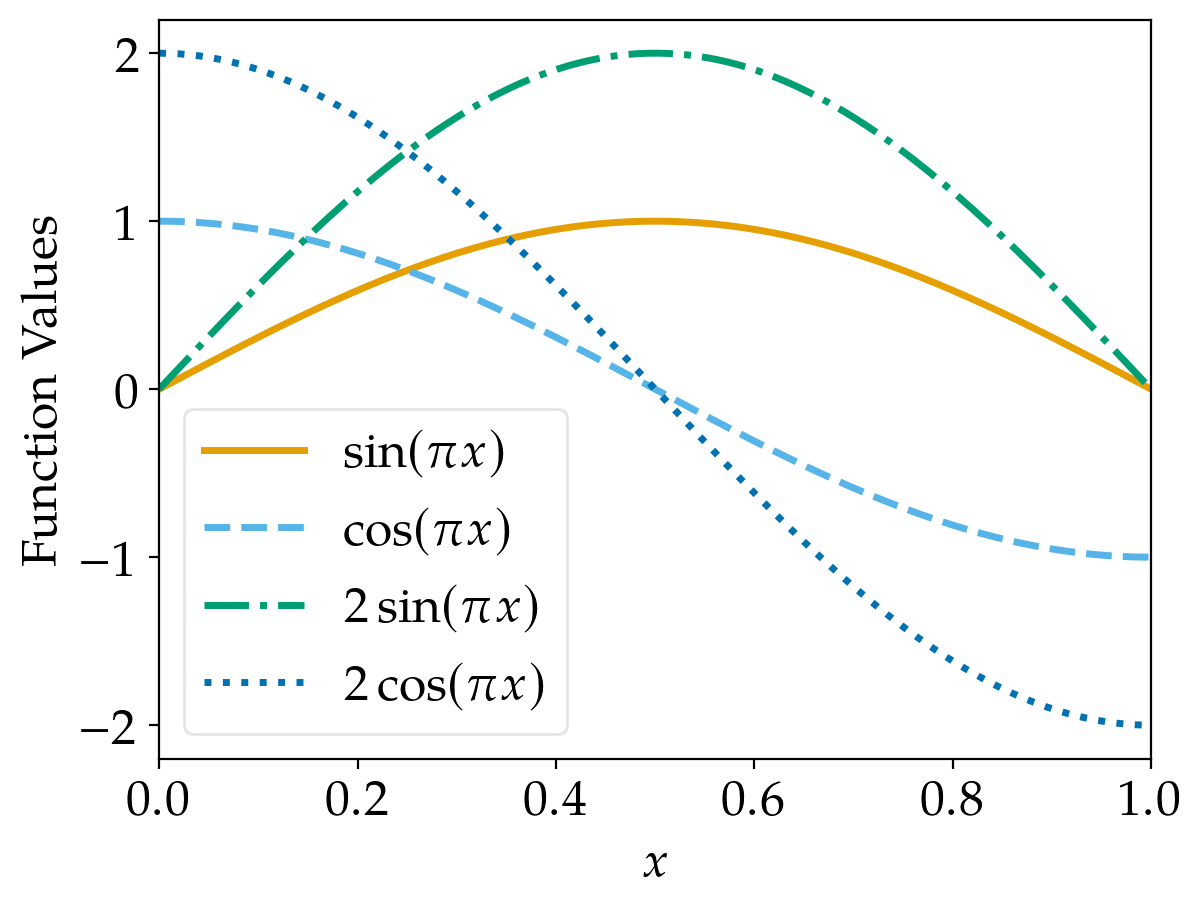The default cycler of matplotlib yields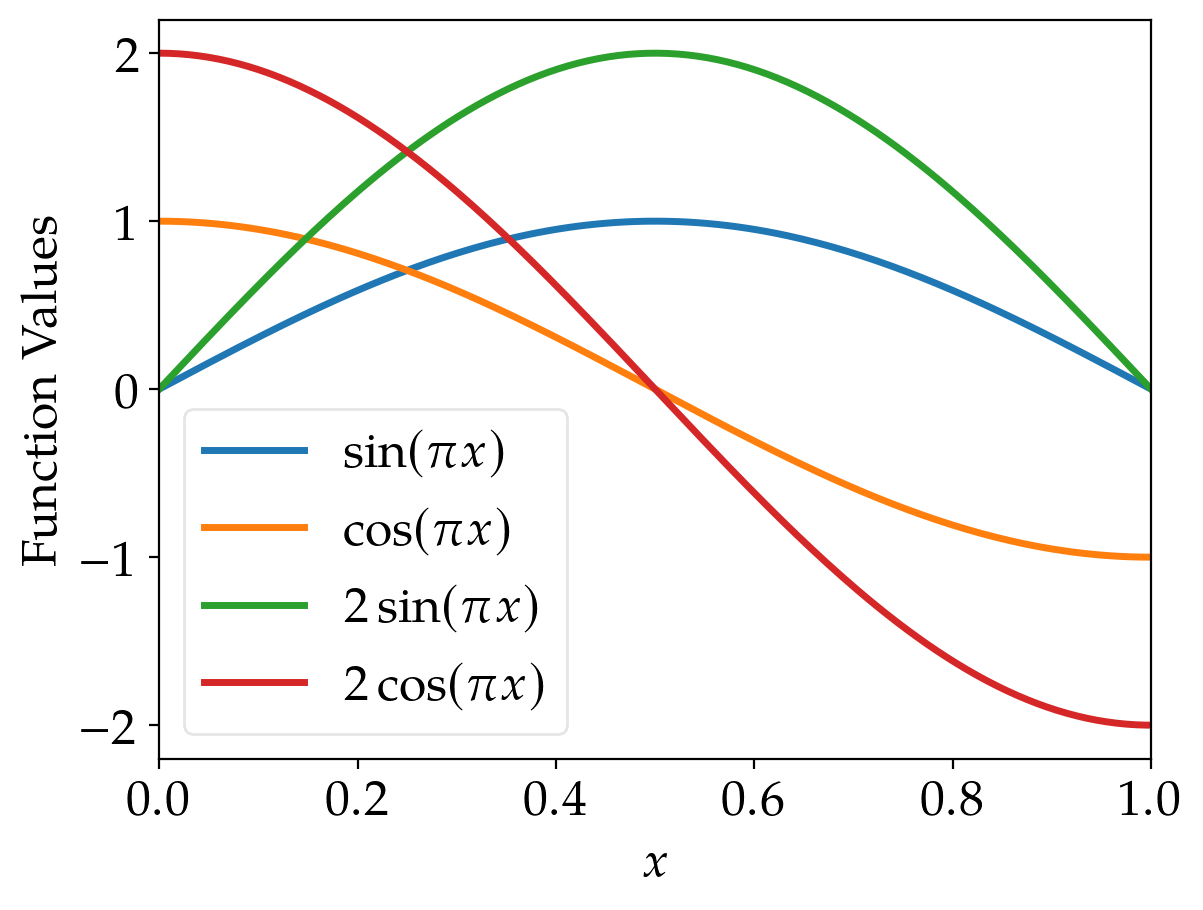These lines are also easily distinguishable (unless you’re red blind; but even in this case, there should be some differences of the brightness, although it is not as easy as for other people). However, if printed in grayscale, it becomes hardly possible to identify the lines correctly using the standard cycler of matplotlib.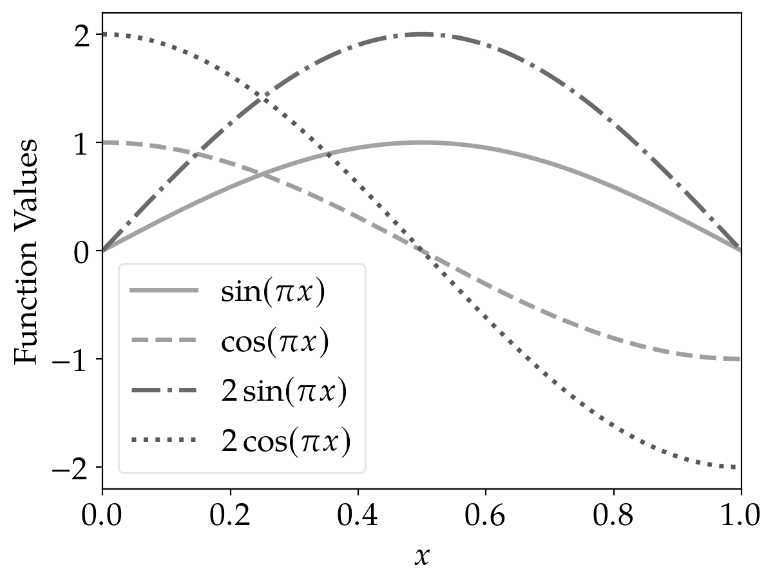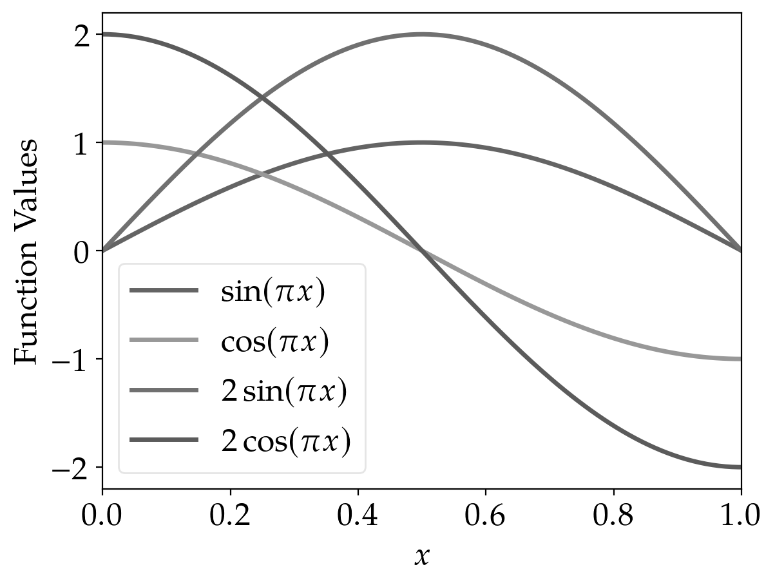line_cycler standard_cycler

The results for scatter plots are similar: Using different marker shapes really helps to identify the plots correctly.

marker_cycler standard_cycler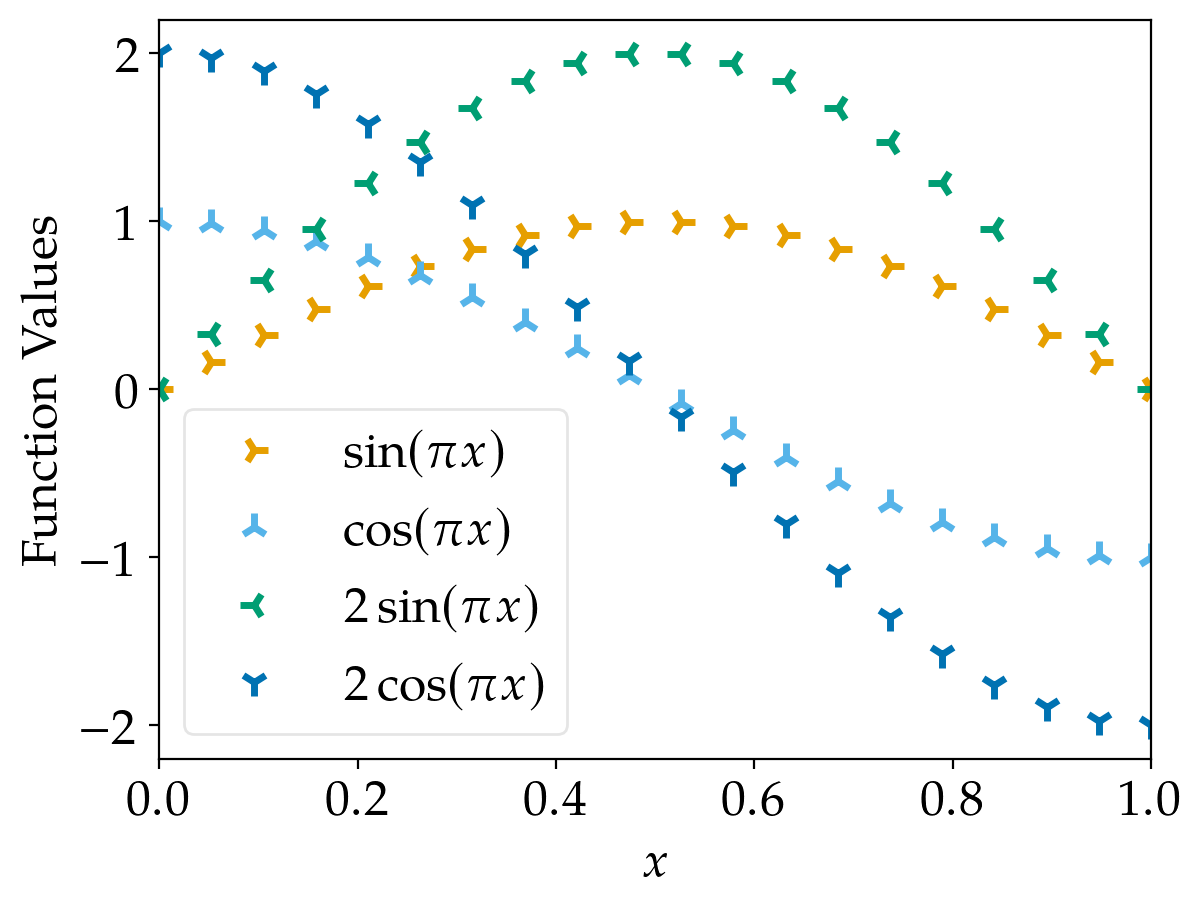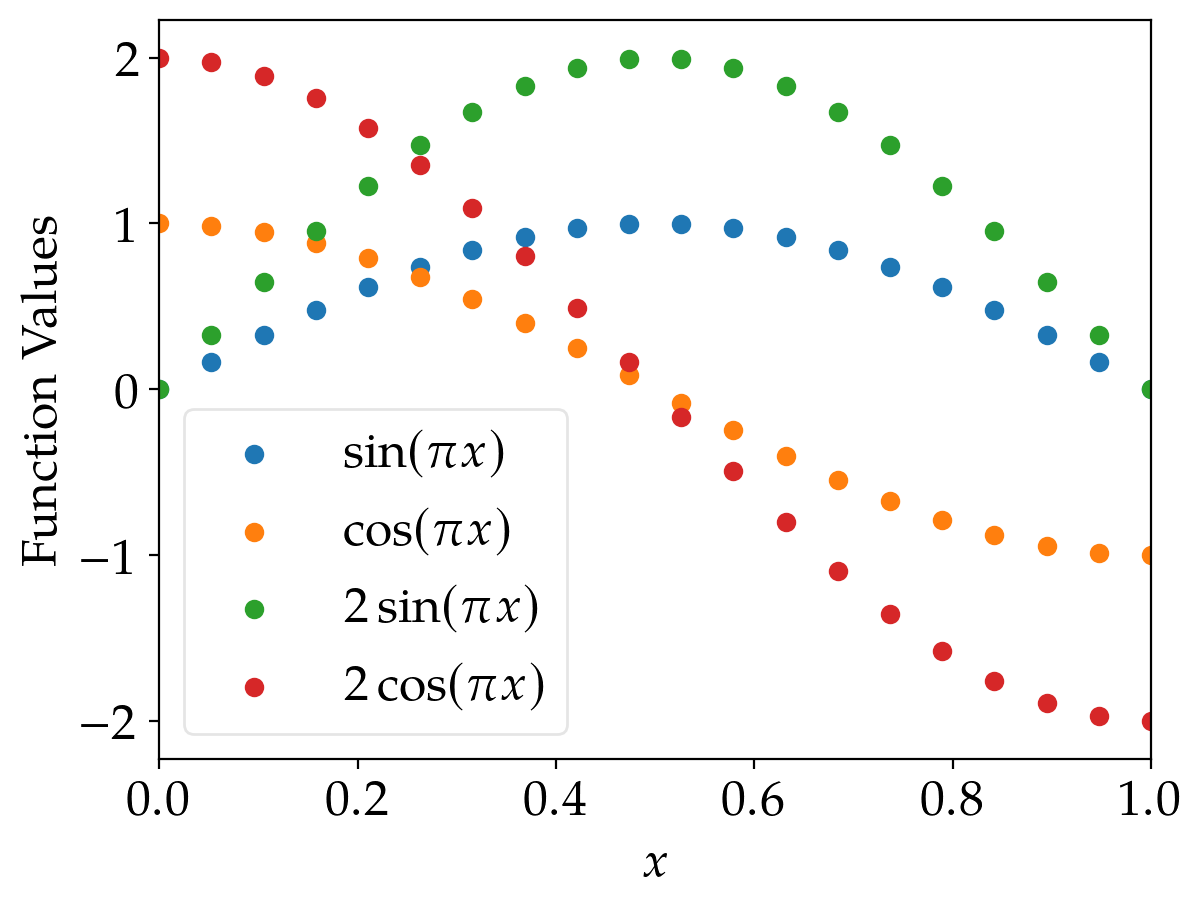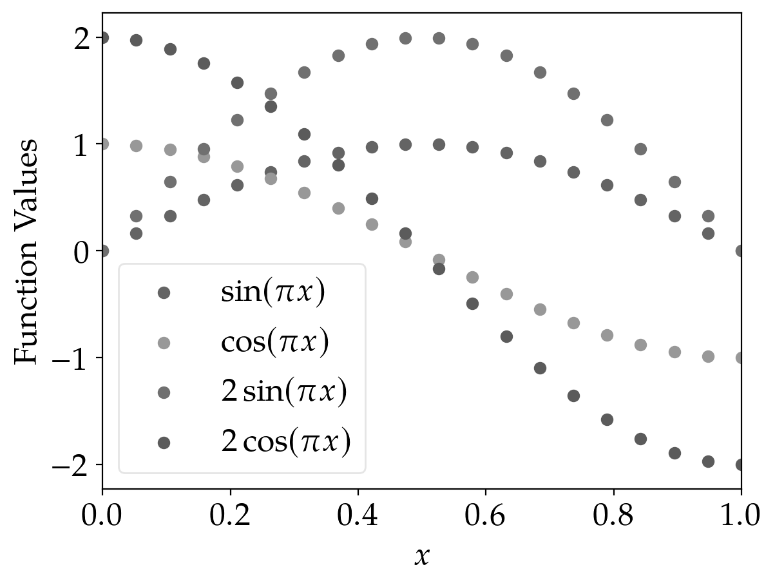In my opinion, it is worth investing some time in creating accessible plots. That’s why I like to use the color blindness simulator to check how figures look like for people affected by some sort of color blindness and in grayscale (monochromatic view). The Python code and Julia code used to produce the figures in this post contain also additional plotting options I like to use. As all code published in this blog, these scripts are released under the terms of the MIT license.

### Python Code

#!/usr/bin/python3

import matplotlib.pyplot as plt

# line cyclers adapted to colourblind people
from cycler import cycler
line_cycler   = (cycler(color=["#E69F00", "#56B4E9", "#009E73", "#0072B2", "#D55E00", "#CC79A7", "#F0E442"]) +
cycler(linestyle=["-", "--", "-.", ":", "-", "--", "-."]))
marker_cycler = (cycler(color=["#E69F00", "#56B4E9", "#009E73", "#0072B2", "#D55E00", "#CC79A7", "#F0E442"]) +
cycler(linestyle=["none", "none", "none", "none", "none", "none", "none"]) +
cycler(marker=["4", "2", "3", "1", "+", "x", "."]))

# matplotlib's standard cycler
standard_cycler = cycler("color", ["#1f77b4", "#ff7f0e", "#2ca02c", "#d62728", "#9467bd", "#8c564b", "#e377c2", "#7f7f7f", "#bcbd22", "#17becf"])

plt.rc("axes", prop_cycle=line_cycler)

plt.rc("text", usetex=True)
plt.rc("text.latex", preamble=r"\usepackage{newpxtext}\usepackage{newpxmath}\usepackage{commath}\usepackage{mathtools}")
plt.rc("font", family="serif", size=18.)
plt.rc("savefig", dpi=200)
plt.rc("legend", loc="best", fontsize="medium", fancybox=True, framealpha=0.5)
plt.rc("lines", linewidth=2.5, markersize=10, markeredgewidth=2.5)

import os
directory = os.path.dirname(os.path.abspath(__file__))

# plots
plt.close("all")

import numpy as np
x = np.linspace(0., 1., 200)

plt.rc("axes", prop_cycle=line_cycler)
plt.figure()
plt.plot(x,   np.sin(np.pi*x), label="$\sin(\pi x)$")
plt.plot(x,   np.cos(np.pi*x), label="$\cos(\pi x)$")
plt.plot(x, 2*np.sin(np.pi*x), label="$2 \sin(\pi x)$")
plt.plot(x, 2*np.cos(np.pi*x), label="$2 \cos(\pi x)$")
plt.legend()
plt.xlabel("$x$")
plt.xlim(x, x[-1])
plt.ylabel("Function Values")
plt.savefig(os.path.join(directory, "line_plot_python.png"), bbox_inches="tight")

plt.rc("axes", prop_cycle=standard_cycler)
plt.figure()
plt.plot(x,   np.sin(np.pi*x), label="$\sin(\pi x)$")
plt.plot(x,   np.cos(np.pi*x), label="$\cos(\pi x)$")
plt.plot(x, 2*np.sin(np.pi*x), label="$2 \sin(\pi x)$")
plt.plot(x, 2*np.cos(np.pi*x), label="$2 \cos(\pi x)$")
plt.legend()
plt.xlabel("$x$")
plt.xlim(x, x[-1])
plt.ylabel("Function Values")
plt.savefig(os.path.join(directory, "line_plot_python_standard.png"), bbox_inches="tight")

import numpy as np
x = np.linspace(0., 1., 20)

plt.rc("axes", prop_cycle=marker_cycler)
plt.figure()
plt.plot(x,   np.sin(np.pi*x), label="$\sin(\pi x)$")
plt.plot(x,   np.cos(np.pi*x), label="$\cos(\pi x)$")
plt.plot(x, 2*np.sin(np.pi*x), label="$2 \sin(\pi x)$")
plt.plot(x, 2*np.cos(np.pi*x), label="$2 \cos(\pi x)$")
plt.legend()
plt.xlabel("$x$")
plt.xlim(x, x[-1])
plt.ylabel("Function Values")
plt.savefig(os.path.join(directory, "marker_plot_python.png"), bbox_inches="tight")

plt.rc("axes", prop_cycle=standard_cycler)
plt.rc("lines", linewidth=1.5, markersize=6, markeredgewidth=1)
plt.figure()
plt.scatter(x,   np.sin(np.pi*x), label="$\sin(\pi x)$")
plt.scatter(x,   np.cos(np.pi*x), label="$\cos(\pi x)$")
plt.scatter(x, 2*np.sin(np.pi*x), label="$2 \sin(\pi x)$")
plt.scatter(x, 2*np.cos(np.pi*x), label="$2 \cos(\pi x)$")
plt.legend()
plt.xlabel("$x$")
plt.xlim(x, x[-1])
plt.ylabel("Function Values")
plt.savefig(os.path.join(directory, "marker_plot_python_standard.png"), bbox_inches="tight")


### Julia Code

import PyPlot; plt = PyPlot
using PyCall
using LaTeXStrings

# line cyclers adapted to colourblind people
cycler = pyimport("cycler").cycler
line_cycler   = (cycler(color=["#E69F00", "#56B4E9", "#009E73", "#0072B2", "#D55E00", "#CC79A7", "#F0E442"]) +
cycler(linestyle=["-", "--", "-.", ":", "-", "--", "-."]))
marker_cycler = (cycler(color=["#E69F00", "#56B4E9", "#009E73", "#0072B2", "#D55E00", "#CC79A7", "#F0E442"]) +
cycler(linestyle=["none", "none", "none", "none", "none", "none", "none"]) +
cycler(marker=["4", "2", "3", "1", "+", "x", "."]))

# matplotlib's standard cycler
standard_cycler = cycler("color", ["#1f77b4", "#ff7f0e", "#2ca02c", "#d62728", "#9467bd", "#8c564b", "#e377c2", "#7f7f7f", "#bcbd22", "#17becf"])

plt.rc("axes", prop_cycle=line_cycler)

plt.rc("text", usetex=true)
plt.rc("text.latex", preamble="\\usepackage{newpxtext}\\usepackage{newpxmath}\\usepackage{commath}\\usepackage{mathtools}")
plt.rc("font", family="serif", size=18.)
plt.rc("savefig", dpi=200)
plt.rc("legend", loc="best", fontsize="medium", fancybox=true, framealpha=0.5)
plt.rc("lines", linewidth=2.5, markersize=10, markeredgewidth=2.5)

directory = @__DIR__

# plots
plt.close("all")

x = range(0., 1., length=200)

plt.rc("axes", prop_cycle=line_cycler)
plt.figure()
plt.plot(x,   sinpi.(x), label=L"$\sin(\pi x)$")
plt.plot(x,   cospi.(x), label=L"$\cos(\pi x)$")
plt.plot(x, 2*sinpi.(x), label=L"$2 \sin(\pi x)$")
plt.plot(x, 2*cospi.(x), label=L"$2 \cos(\pi x)$")
plt.legend()
plt.xlabel(L"$x$")
plt.xlim(x, x[end])
plt.ylabel("Function Values")
plt.savefig(joinpath(directory, "line_plot_julia.png"), bbox_inches="tight")

plt.rc("axes", prop_cycle=standard_cycler)
plt.figure()
plt.plot(x,   sinpi.(x), label=L"$\sin(\pi x)$")
plt.plot(x,   cospi.(x), label=L"$\cos(\pi x)$")
plt.plot(x, 2*sinpi.(x), label=L"$2 \sin(\pi x)$")
plt.plot(x, 2*cospi.(x), label=L"$2 \cos(\pi x)$")
plt.legend()
plt.xlabel(L"$x$")
plt.xlim(x, x[end])
plt.ylabel("Function Values")
plt.savefig(joinpath(directory, "line_plot_julia_standard.png"), bbox_inches="tight")

x = range(0., 1., length=20)

plt.rc("axes", prop_cycle=marker_cycler)
plt.figure()
plt.plot(x,   sinpi.(x), label=L"$\sin(\pi x)$")
plt.plot(x,   cospi.(x), label=L"$\cos(\pi x)$")
plt.plot(x, 2*sinpi.(x), label=L"$2 \sin(\pi x)$")
plt.plot(x, 2*cospi.(x), label=L"$2 \cos(\pi x)$")
plt.legend()
plt.xlabel(L"$x$")
plt.xlim(x, x[end])
plt.ylabel("Function Values")
plt.savefig(joinpath(directory, "marker_plot_julia.png"), bbox_inches="tight")

plt.rc("axes", prop_cycle=standard_cycler)
plt.rc("lines", linewidth=1.5, markersize=6, markeredgewidth=1)
plt.figure()
plt.scatter(x,   sinpi.(x), label=L"$\sin(\pi x)$")
plt.scatter(x,   cospi.(x), label=L"$\cos(\pi x)$")
plt.scatter(x, 2*sinpi.(x), label=L"$2 \sin(\pi x)$")
plt.scatter(x, 2*cospi.(x), label=L"$2 \cos(\pi x)$")
plt.legend()
plt.xlabel(L"$x$")
plt.xlim(x, x[end])
plt.ylabel("Function Values")
plt.savefig(joinpath(directory, "marker_plot_julia_standard.png"), bbox_inches="tight")


Tags:

Categories:

Updated: